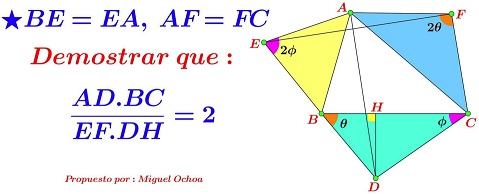# A Problem with Two Isosceles Triangles

### Source

Leo Giugiuc has kindly posted at the CutTheKnotMath facebook page another problem by Miguel Ochoa Sanchez and a solution by Claudia Nanuti, Diana Trailescu, Dan Sitaru and Leo Giugiuc:### Problem

Given two angles $\varphi,\theta\le 90^{\circ}$ and $\Delta ABC.$ On the sides of the latter erect Two Isosceles Triangless $ACF\;AF=AC$ and $ABE\;AE=BE,$ and $\Delta BCD$ such that $\angle BCD=\varphi,$ $\angle CBD=\theta,$ $\angle AFC=2\theta,$ $\angle AEB=2\varphi.$ Finally, let $H$ be the foot of the perpendicular from $D$ to $BC.$Prove that

$\displaystyle\frac{AD\cdot BC}{EF\cdot DH}=2.$

### Solution

Solution employs complex numbers.

We choose, as usual, $A=i,$ $B=-b,$ and $C=c,$ $b,c\gt 0.$ Without loss of generality we may assume $b\lt c.\;$ Let $D=a-di,\;$ $d\gt 0.\;$ Since $\theta$ and $\varphi$ are acute, clearly, $b\lt a\lt c.\;$

We are going to use the following trigonometric lemma that is easily proved with the addition and subtraction formulas.

For a real number $t,$

$\cos 2t+i\sin 2t -1=2i(\cos t+i\sin t)\cdot\sin t.$

To continue, we get a sequence of equivalent identities:

\displaystyle\begin{align}\frac{A-E}{B-E}&=\cos 2\varphi +i\sin 2\varphi ,\\ \frac{i-E}{B-E}&=\cos 2\varphi +i\sin 2\varphi ,\\ \frac{i-E}{-b-i}&=\frac{\cos 2\varphi +i\sin 2\varphi}{1-(\cos 2\varphi +i\sin 2\varphi)} ,\\ \frac{i-E}{-b-i}&=-\frac{\cos 2\varphi +i\sin 2\varphi}{2i(\cos\varphi +i\sin\varphi)\sin\varphi} ,\\ &=\frac{i(\cot\varphi +i)}{2},\\ &=\frac{i(c-a+id)}{2d}. \end{align}

From here we obtain

$\displaystyle E=\frac{a-c-bd+i(bc-ab+d)}{2d}.$

Similarly,

$\displaystyle F=\frac{a+b+cd+i(ac+bc+d)}{2d}.$

Thus, $AD=\sqrt{a^2+(d+1)^2},$ $BC=b+c,$ $DH=d,$ and $EF=\displaystyle\frac{b+c}{2d}=\sqrt{a^2+(d+1)^2}$ so that indeed $\displaystyle\frac{AD\cdot BC}{EF\cdot DH}=2.$

Note that if we used slopes, we would easily prove that $AD\perp EF.$